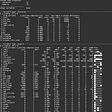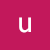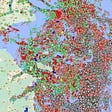# Predicting Victorian State Elections: STRONG Transition Model

• The UNS model:
• The Transition model:
`import pandas as pd pd.plotting.register_matplotlib_converters()import matplotlib.pyplot as pltfrom sklearn.metrics import mean_absolute_errordata2018 = pd.read_csv('~/Desktop/WORK/Python/Vic_Election/data/2pp2018.csv')data2014 = pd.read_csv('~/Desktop/WORK/Python/Vic_Election/data/2pp2014.csv')def clean_voteshare(n):    n = list(n)    n.remove('%')    n = ''.join(n)    n = float(n)    return nfor x in range(0, len(data2014.index)):    data2014.loc[x, 'ALP_share'] = clean_voteshare(data2014.loc[x, 'ALP_share'])    data2018.loc[x, 'ALP_share'] = clean_voteshare(data2018.loc[x, 'ALP_share'])    data2014.loc[x, 'LNP_share'] = clean_voteshare(data2014.loc[x, 'LNP_share'])    data2018.loc[x, 'LNP_share'] = clean_voteshare(data2018.loc[x, 'LNP_share'])parties = ['ALP', 'LNP']# Set threshold and then get lists for statewide support and strong support of each partythresh = 20Vs =  {}V = {}      for party in parties:        num = 0    for x in range(0, len(data2014.index)):        num += data2014.loc[x, 'Total votes']*data2014.loc[x, party+'_share']            V[party] = num/sum(list(data2014['Total votes']))for party in parties:        num = 0    for x in range(0, len(data2014.index)):        num += data2014.loc[x, 'Total votes']*max(data2014.loc[x, party+'_share'] - thresh, 0)         Vs[party] = num/sum(list(data2014['Total votes']))# Candidate P's:# P = {'ALP': 57.3, 'LNP': 42.7}, actual election result, i.e. polls accurate # P = {'ALP': 55, 'LNP': 45}, YouGov 24th Nov 2018P = {'ALP': 55, 'LNP': 45} # Can change this as needed Pw = {}Ps = {}# Here I set up the the predicted strong and weak support for each party.for party in parties:        Pw[party] = max(P[party] - Vs[party], 0)    Ps[party] = min(P[party], Vs[party])###################################################################V_splits = {} for x in Vs.keys():     V_splits[x+'_strong'] = Vs[x]    V_splits[x+'_weak'] = V[x] - Vs[x]        P_splits = {}for x in Pw.keys():     P_splits[x+'_weak'] = Pw[x]for x in Ps.keys():    P_splits[x+'_strong'] = Ps[x]data2014['ALP_strong'] = 0for x in range(0, len(data2014.index)):    data2014.loc[x, 'ALP_strong'] = max(data2014.loc[x, 'ALP_share'] - thresh,0)  data2014['ALP_weak'] = data2014['ALP_share'] - data2014['ALP_strong']data2014['LNP_strong'] = 0for x in range(0, len(data2014.index)):    data2014.loc[x, 'LNP_strong'] = max(data2014.loc[x, 'LNP_share'] - thresh,0)  data2014['LNP_weak'] = data2014['LNP_share'] - data2014['LNP_strong']   # From here on out we are just implementing the transition model on our extended party list# since I am lazy and this is mostly the same as the code in the transition model # article, I have been very lax with commenting here (sorry, I know it's bad).  total_share = 0for x in V_splits.keys():    total_share += max(P_splits[x] - V_splits[x], 0)    share = {}for x in V_splits.keys():    share[x] = max(P_splits[x] - V_splits[x], 0)/total_share       class electorate(object):        def __init__(self, C, name):        self.C = C        self.name = name            def A(self, party):        if P_splits[party] - V_splits[party] < 0:            return self.C[party] * (P_splits[party]/V_splits[party])        else:            return self.C[party] + share[party]*SwingVoters[self.name]           districts = []for x in range(0, len(data2014.index)):    district = []    district.append(data2014.loc[x, 'District'])    district.append(data2014.loc[x, 'ALP_strong'])    district.append(data2014.loc[x, 'ALP_weak'])    district.append(data2014.loc[x, 'LNP_strong'])    district.append(data2014.loc[x, 'LNP_weak'])        districts.append(district)        SwingVoters = {}district_objs = {}for x in districts:    C= {'ALP_strong': x, 'ALP_weak': x, 'LNP_strong': x, 'LNP_weak': x}    swingvoters = 0    for party in V_splits.keys():        swingvoters += C[party] * max(1-P_splits[party]/V_splits[party] ,0)            SwingVoters[x] = swingvoters        district_objs[x] = electorate(C, x)preds_alp = {} preds_lib = {}   for x in district_objs.keys():    preds_alp[x] = district_objs[x].A('ALP_strong') + district_objs[x].A('ALP_weak')    preds_lib[x] = district_objs[x].A('LNP_strong') + district_objs[x].A('LNP_weak')      real_alp = {}real_lib = {}for x in range(0, len(data2018.index)):    district = data2014.loc[x, 'District']    real_alp[district] = data2018.loc[x, 'ALP_share']    real_lib[district] = data2018.loc[x, 'LNP_share']    d = {'electorate': list(district_objs.keys()), 'alp_real':list(real_alp.values()),     'alp_preds': list(preds_alp.values()), 'lib_real': list(real_lib.values()),     'lib_preds': list(preds_lib.values())}df = pd.DataFrame(data=d)lineStart = df.alp_real.min()lineEnd = df.alp_real.max()fig = plt.figure(dpi=800)plt.figure()plt.scatter(x=df['alp_preds'], y=df['alp_real'], color='r', marker = '2')plt.scatter(x=df['lib_preds'], y=df['lib_real'], color='b', marker = '2')plt.plot([lineStart, lineEnd], [lineStart, lineEnd], 'k-', color = 'black')plt.plot([50, 50], [lineStart, lineEnd], color='black', linestyle='dashed', linewidth=1)plt.plot([lineStart, lineEnd], [50, 50], color='black', linestyle='dashed', linewidth=1)plt.xlim(lineStart, lineEnd)plt.ylim(lineStart, lineEnd)plt.xlabel('Predicted  Voteshare')plt.ylabel('Actual Voteshare')plt.title('STRONG Model Accuracy for 2PP Voteshare in 2018')#plt.savefig('accuracy_Transition.pdf')plt.show()# Calculating the MAE# (since we are calculating this for 2PP votes where party = 100 - party# the MAE will be the same regardless of whether we choose alp or lib data to compare)MAE = mean_absolute_error(df['alp_real'], df['alp_preds'])print('The MAE of predictions is: ' + str(MAE))# Getting a list of incorrect predictions:    alp_fp = []alp_fn = []for x in range(0, len(df.index)):        if df.loc[x, 'alp_real'] < 50 and df.loc[x, 'alp_preds'] >= 50:        alp_fp.append(df.loc[x,'electorate'])            if df.loc[x, 'alp_real'] >= 50 and df.loc[x, 'alp_preds'] < 50:        alp_fn.append(df.loc[x,'electorate'])print('False positives for the ALP/ False negatives for LNP were: ')print(alp_fp)print()print('False negatives for the ALP/ False positives for the LNP were: ')print(alp_fn)# Finally, let's look at the total predicted ALP seats v actual ALP seats:alp_wins = []alp_pred_wins = []    lib_wins = []lib_pred_wins = []for x in range(0, len(df.index)):        if df.loc[x, 'alp_real'] > 50:        alp_wins.append(df.loc[x,'electorate'])    else:        lib_wins.append(df.loc[x,'electorate'])            if df.loc[x, 'alp_preds'] > 50:        alp_pred_wins.append(df.loc[x,'electorate'])            else:        lib_pred_wins.append(df.loc[x,'electorate'])    print('The ALP was predicted to win: ' + str(len(alp_pred_wins)) + ' seats')print('They actually won: ' + str(len(alp_wins)) + ' seats')print()print('The LNP was predicted to win: ' + str(len(lib_pred_wins)) + ' seats')print('They actually won: ' + str(len(lib_wins)) + ' seats')`

--

--

--

Love podcasts or audiobooks? Learn on the go with our new app.

## A Swift look at Taylor’s music over the years## Analysis of a Synthetic Breast Cancer Dataset in R## The Need for efficient Data Science Customers Requests## Social Network Analysis Web App## Working with HDF5 files and creating CSV files## A look at the US and Israel’s special relationship with regard to the Coronavirus## Human Pose Estimation : Simplified## Improving Data Literacy at GoCardless## Ghosts of Future Past## Biodiversity talks in Geneva need more talk## The Curse of Variability## Redberry Lake Week 2- Muskeg Lake Cree Nation. Bedfilling via Hugelkultur method.